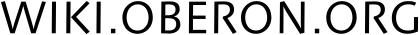#### Инструменты сайта

ob:visual:math:en

Module Math is a basic library for numerical computations. It offers the most frequently used functions and constants.

PROCEDURE Pi (): REAL

Returns an approximation of the value of pi.

Returns the value of the 'angle' in radians.

PROCEDURE Random (): REAL;

Returns random number from 0.0 to 1.0.

* Powers and logarithms *

PROCEDURE Sqrt (x: REAL): REAL

Returns the square root of x. Precondition: x >= 0.0

PROCEDURE Exp (x: REAL): REAL

Returns e^x.

PROCEDURE Ln (x: REAL): REAL

Returns the natural logarithm of x. Precondition: x >= 0.0

PROCEDURE Log (x: REAL): REAL

Returns the logarithm to the basis 10 of x. Precondition: x >= 0.0

PROCEDURE Power (x, y: REAL): REAL

Returns x^y. Precondition: x >= 0.0

PROCEDURE IntPower (x: REAL; n: INTEGER): REAL

Returns x^n. IntPower(0, 0) gives 1.

* Trigonometric and hyperbolic functions *

The arguments for all trigonometric and hyperbolic functions must be given in radians, and the inverse trigonometric and hyperbolic functions are calculated in radians (1 radian = 180/pi degrees).

PROCEDURE Sin (x: REAL): REAL

Returns the sine of x. Postcondition: -1.0 <= result <= 1.0

PROCEDURE Cos (x: REAL): REAL

Returns the cosine of x. Postcondition: -1.0 <= result <= 1.0

PROCEDURE Tan (x: REAL): REAL

Returns the tangent of x.

PROCEDURE ArcSin (x: REAL): REAL

Returns the arcus sine of x. Precondition: -1.0 <= x <= 1.0 Postcondition: -pi/2.0 <= result <= pi/2.0

PROCEDURE ArcCos (x: REAL): REAL

Returns the arcus cosine of x. Precondition: -1.0 <= x <= 1.0 Postcondition: 0.0 <= result <= pi

PROCEDURE ArcTan (x: REAL): REAL

Returns the arcus tangent of x. Postcondition: -pi/2.0 <= result <= pi/2.0

PROCEDURE ArcTan2 (y, x: REAL): REAL

Returns the argument (angle) of the complex number x + iy measured anti-clockwise from the positive real axis. Postcondition: -pi < result <= pi

PROCEDURE Sinh (x: REAL): REAL

Returns the hyperbolic sine of x.

PROCEDURE Cosh (x: REAL): REAL

Returns the hyperbolic cosine of x. Postcondition: 1.0 <= result

PROCEDURE Tanh (x: REAL): REAL

Returns the hyperbolic tangent of x. Postcondition: -1.0 <= result <= 1.0

PROCEDURE ArcSinh (x: REAL): REAL;

Returns the inverse hyperbolic sine of x.

PROCEDURE ArcCosh (x: REAL): REAL;

Returns the inverse hyperbolic cosine of x. Precondition: 1.0 <= x Postcondition: 0.0 <= result

PROCEDURE ArcTanh (x: REAL): REAL;

Returns the inverse hyperbolic tangent of x. Precondition: -1.0 <= x <= 1.0

* Miscellaneous functions *

PROCEDURE Sign (x: REAL): REAL

Returns the sign um function of x, that is 1.0 if x > 0.0, -1.0 if x < 0.0 and 0.0 with the sign of x if x = 0.0. Postcondition: result IN {-1.0, 0.0, 1.0}

PROCEDURE Floor (x: REAL): REAL

Returns the greatest integer less than or equal to x. Identical to FLOOR(x).

PROCEDURE Ceiling (x: REAL): REAL

Returns the smallest integer greater than or equal to x.

PROCEDURE Trunc (x: REAL): REAL

Trunc truncates its argument to the next nearest integer towards zero.

PROCEDURE Round (x: REAL): REAL

Same as Floor(x + 0.5).

ob/visual/math/en.txt · Последнее изменение: 2022/10/02 20:37 — iadenisov

### Инструменты страницы